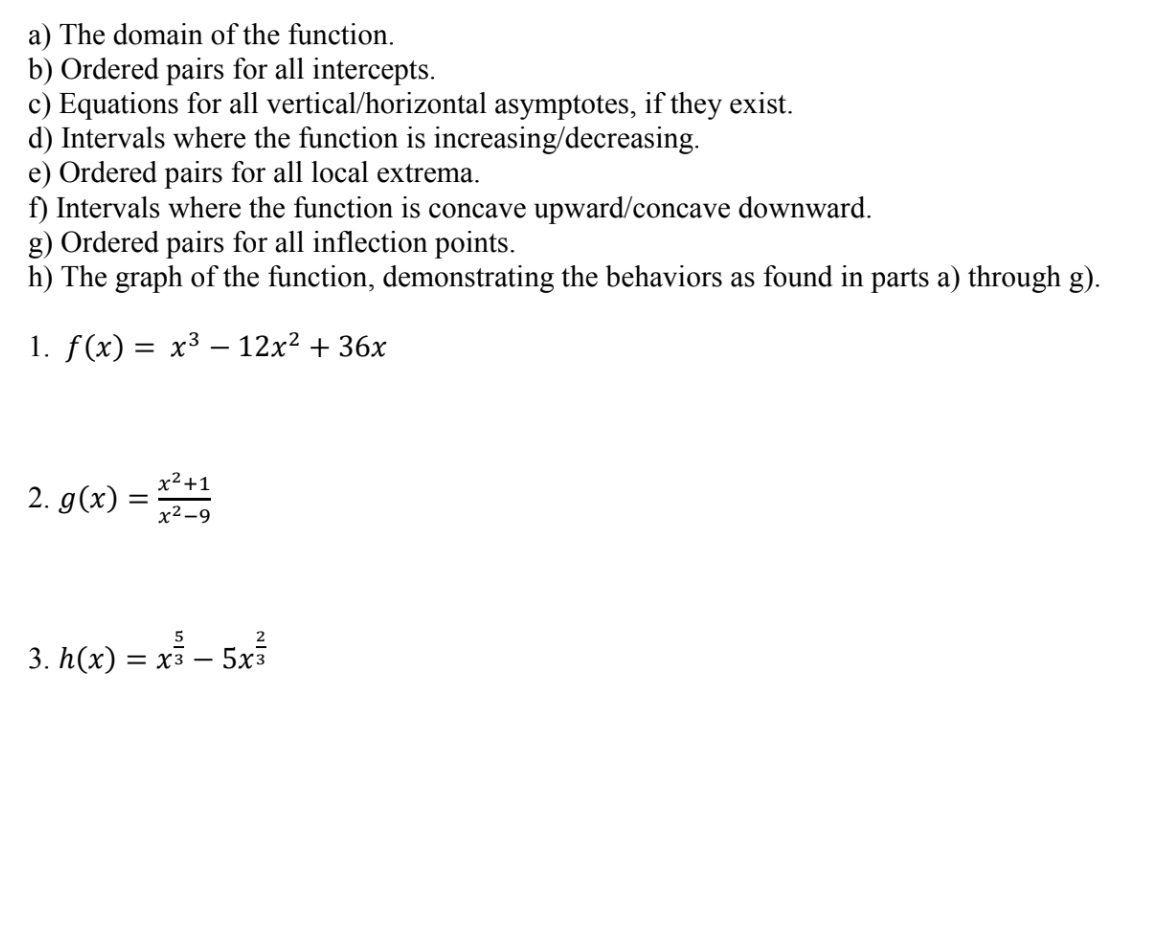# a) The domain of the function.b) Ordered pairs for all intercepts.c) Equations for all vertical/horizontal asymptotes, if they existd) Intervals where the function is increasing/decreasinge) Ordered pairs for all local extremaft) Intervals where the function is concave upward/concave downwardg) Ordered pairs for all inflection points.h) The graph of the function, demonstrating the behaviors as found in parts a) through g)I. f(x)-x3-12x2 + 36X2x2-9523. h(x) -xs - 5x3

Question
1 views

solve #2, g to hhelp_outlineImage Transcriptionclosea) The domain of the function. b) Ordered pairs for all intercepts. c) Equations for all vertical/horizontal asymptotes, if they exist d) Intervals where the function is increasing/decreasing e) Ordered pairs for all local extrema ft) Intervals where the function is concave upward/concave downward g) Ordered pairs for all inflection points. h) The graph of the function, demonstrating the behaviors as found in parts a) through g) I. f(x)-x3-12x2 + 3 6X 2 x2-9 5 2 3. h(x) -xs - 5x3 fullscreen
check_circle

Step 1

Differentiate g(x) with respect to x.

Step 2

Differentiate g'(x) with respect to x.

Step 3

Equate the second derivative g...

### Want to see the full answer?

See Solution

#### Want to see this answer and more?

Solutions are written by subject experts who are available 24/7. Questions are typically answered within 1 hour.*

See Solution
*Response times may vary by subject and question.
Tagged in
MathCalculus

### Functions